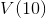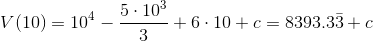# Calculus 1 : How to find position

## Example Questions

← Previous 1 3 4 5 6 7 8 9 17 18

### Example Question #1 : How To Find Position

Find a vector perpendicular to (4,3).

(-4,3)
(8,6)
(-4,-3)
(3,-4)
(4,-3)
Explanation:

In general, if we have a vector (a,b), a perpendicular vector is (b,-a).

So here, the perpendicular vector is (3,-4).

### Example Question #1 : How To Find Position

if a=i + 2j - 3k and b=4i + 7k, express the vector 3a + 2b.

14i + 4j + 15k
10i + 5j + 6k
12i - 6j + 6k
2i + 4j - 6k
11i + 6j + 5k
Correct answer: 11i + 6j + 5k
Explanation:

To express the vector in terms of i, j, and k, we need to combine like terms and distribute.

3a + 2b

= 3(i + 2j - 3k) + 2(4i + 7k)

= 3i + 6j - 9k + 8i + 14k

= 11i +6j + 5k

### Example Question #3 : How To Find Position

The velocity of a particle is given by the function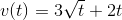.  What is it's position at time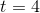if it's starting position was 4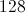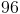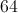Explanation:

To find the position from velocity, the function must be integrated.  This gives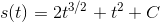.  substituting 4 forand using the given initial condition gives the answer

### Example Question #4 : How To Find Position

The veloctiy of a particle at timeis given by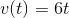.  What is its change in position between time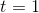and time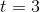?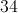Cannot be determined.Explanation:

The position function is the intergral of the velocity function.  So here, position is given by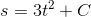whereis the constant of integration.  Because only a difference in position is asked, and not an absolute position, the constant of integration cancels out.

### Example Question #5 : How To Find Position

Find the position at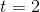if the acceleration function is: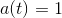.Explanation:

To find the position from the acceleration function, integrate the acceleration function twice.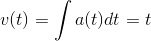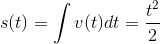Substituteto find the postion.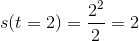### Example Question #6 : How To Find Position

Find the position atif the acceleration is: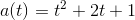.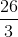Explanation:

To find the position function, integrate the acceleration function twice.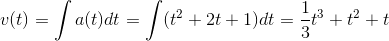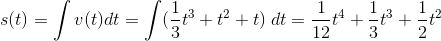Evalute the position at.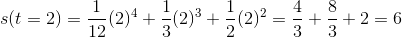### Example Question #7 : How To Find Position

The velocity of an object is given by the following equation: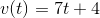If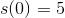, find the equation for the position of the object at any time.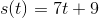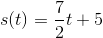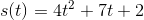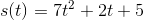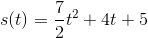Explanation:

Velocity is the derivative of position, so in order to obtain an equation for position, we must integrate the given equation for velocity: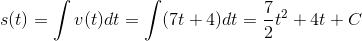The next step is to solve for C by applying the given initial condition, s(0)=5: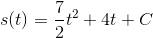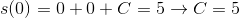So our final equation for position is:### Example Question #8 : How To Find Position

The position function of a ball from the ground when it is thrown by a pitcher is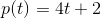.

Where is the ball located at?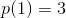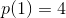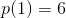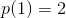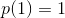Explanation:

To find the position of the ball, we plug inSoturns into: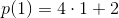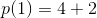### Example Question #9 : How To Find Position

Function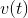gives the velocity of a particle as a function of time.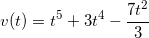Find the equation that models the particle's postion as a function of time.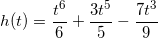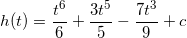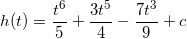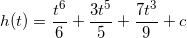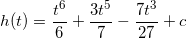Explanation:

Recall that velocity is the first derivative of position, and acceleration is the second derivative of position. We begin with velocity, so we need to integrate to find position and derive to find acceleration.

We are starting with the followingWe need to perform the following: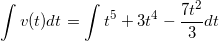Recall that to integrate, we add one to each exponent and divide by the that number, so we get the following. Don't forget your +c as well.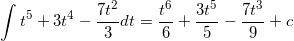Which makes our position function, h(t), the following:### Example Question #10 : How To Find Position

Consider the velocity function modeled in meters per second by v(t).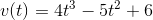Find the position of a particle whose velocity is modeled byafterseconds.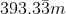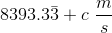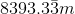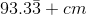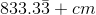Explanation:

Recall that velocity is the first derivative of position, so to find the position function we need to integrate.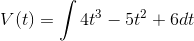Becomes,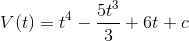Then, we need to find